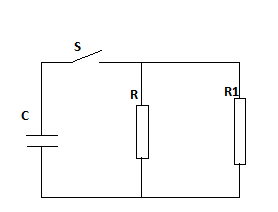# What is the time constant for the discharge of the capacitor in the figure below

What is the time constant for the discharge of the capacitor in the figure below when C=7 μF,R=4 kΩ,R1=1.0 kΩC=7 μF,R=4 kΩ,R1=1.0 kΩ?